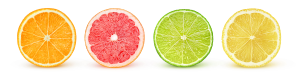# Row in Math

## What Is a Row in Math?

A row is simply the horizontal arrangement of objects, side by side, from left to right. It could be people, numbers, or others, placed alongside each other.

Have you observed how the desks and chairs are arranged in a classroom? How does the seating arrangement work? Well, students sit in “rows!”

Let’s see some examples.

Example 1: Children sit in 2 rows. Each row has 4 children.

Example 2:

A single row of 5 numbers: 45, 12, 56, 88, 121

## Definition of Row

A row can be defined as an arrangement of objects or data in a horizontal line from left to right.

Example 1: A single row of flowers containing 7 flowers.

Example 2: The donuts in the given image are arranged in 3 rows and each row has four donuts.

Note: A common term that goes along with “row” is “column.” Column refers to vertical arrangements of objects, from top to bottom.

## Finding the Total Number of Items in the Given Rows

To find the number of items in a given row, we multiply the number of rows with the number of items in each row.

For example,

Number of stars in each row = 4

Number of rows $= 3$

So, the total number of stars is $4 \times 3 = 12$

## Applications of Row in Math

A row in math has some significant mathematical applications.

• Solving matrices: Matrix is an arrangement of numbers into rows and columns.
• Spreadsheets: we use these while working on computers using rows and columns structure.
• Arrays: An array is a collection of objects in rows and columns. Arrays represent multiplication and division problems visually. Rows represent the number of groups and columns represent the size of each group.

## Conclusion

In this article, we learned about rows and saw them visually. Rows have many real-life and mathematical applications. Let’s solve some interesting examples.

## Solved Examples

1. How many rows are there in the given picture? How many oranges are there in each row?

Solution:

There is a single row of apples in the given picture. There are 5 apples arranged in a row.

2. How many toy cars are arranged in the given row?

Solution:

There are 5 cars in the given row.

3. Determine the number of pairs of shoes in the given row.

Solution:

There are 4 pairs of shoes in the given row.

4. How many cupcakes are there in the given image?

Solution:

There are 4 rows of cupcakes in the given image.

Number of cupcakes in each row $= 4$

Total number of cupcakes $= 4 \times 4 = 16$

5. How many rows and flowers are there in the given image?

Solution:

Number of roses in one row $= 4$

Number of rows $= 3$

Total roses $= 4 \times 3 = 12$

## Practice Problems

1

### Find the rows in the given figure.4
1
2
0
CorrectIncorrect
There is one row of lemon slices.
2

### Count the number of jars of smoothies in the given row.1
6
5
2
CorrectIncorrect
There are 6 jars of smoothies placed in a single row.
3

diagonal
vertical
horizontal
reverse
CorrectIncorrect